# LSC and Friends of Northside Meetings

Attending an LSC or FON meeting is a great way to stay on top of what's happening at school. They're open to all parents and guardians. We look forward to your attendance and participation!

# Local School Council (LSC)

Tuesday, September 17
6:30 pm
Faculty Lounge

The Local School Council (LSC) - the voice in the continuing evolution of Northside - is an elected body that plays an important role in the decision-making that affects our school. The LSC is responsible for approving the Continuous Improvement Work Plan (CIWP), principal selection and evaluation, and allocating discretionary funds to school programs.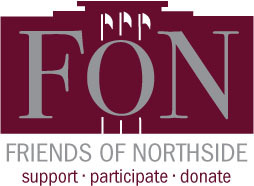# Friends of Northside

Tuesday, September 24
7:00 pm
Faculty Lounge

Friends of Northside (FON) is the “one-stop-shop” for NCP families to get involved. FON facilitates communication between parents and the school, organizes parent volunteers, plans and sponsors social/educational events, and raises much-needed funds to bridge the gap due to CPS budget cuts. FON supports the school’s mission to maintain and enhance the learning experience for all students of Northside College Prep High School.

To find out more about Friends of Northside, please visit their website HERE.

 table div table+table+table+table+table div table{width:100%;padding:0}table div table+table+table+table+table div table img{width:96.23%;padding:0;float:none}table div table+table+table+table+table div table td{width:100%;padding:0 1.88% 18px}/* styles */# Host Families Wanted

Wednesday, October 9-Friday, October 18

Northside College Prep will be hosting 12 students from Prague from October 9-18. We are looking for NCP families, preferably with a sophomore, junior, or senior, willing to host a student during that time and show them some of the amazing things that Northside and Chicago have to offer. If you are interested in hosting, please fill out an application form HERE. Information about the trip can be found in this Prague FAQ document. For additional information, please contact assistant principal Barry Smith.

 table div table+table+table+table+table+table+table div table{width:100%;padding:0}table div table+table+table+table+table+table+table div table img{width:96.23%;padding:0;float:none}table div table+table+table+table+table+table+table div table td{width:100%;padding:0 1.88% 18px}/* styles */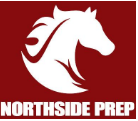# NCP Athletic Boosters Meeting

Thursday, September 19
7:00 pm
Faculty Lounge

The NCP Athletic Boosters seek to organize support and assistance for our exciting athletic programs, their coaches and our amazing student athletes! Please join us to share your ideas and concerns and help us in our quest to increase attendance and student participation at our team events. Get involved and let’s celebrate the incredible achievements of our students outside of the classroom!

All parents and guardians are welcome. Go Mustangs!!!

 table div table+table+table+table+table+table+table+table+table div table{width:100%;padding:0}table div table+table+table+table+table+table+table+table+table div table img{width:96.23%;padding:0;float:none}table div table+table+table+table+table+table+table+table+table div table td{width:100%;padding:0 1.88% 18px}/* styles */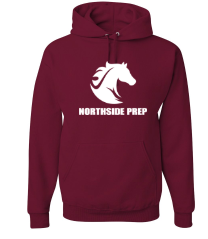# Northside Mustangs Spirit Wear Store

The Athletic Boosters have coordinated with All Seasons Screen Printing & Embroidery again this year to make NCP Spirit wear available to everyone online. The new mustang logo has now been incorporated into the offerings from last year. Shop for the latest gear HERE.

The store will be open until 11:59 pm on Sunday, September 29. Get your spirit wear today!

 table div table+table+table+table+table+table+table+table+table+table+table div table{width:100%;padding:0}table div table+table+table+table+table+table+table+table+table+table+table div table img{width:96.23%;padding:0;float:none}table div table+table+table+table+table+table+table+table+table+table+table div table td{width:100%;padding:0 1.88% 18px}/* styles */# Parking Lot Safety

Our NCP school parking lot is a bustling place before and after school. Families dropping off and picking up students are asked to be mindful of both safety and courtesy. Please assist us by ensuring that traffic lanes stay clear during drop-off and pick-up times and by following the parking lot procedures.

# Parking Lot Procedures

ALL vehicles MUST exit onto Kedzie. You may enter the parking lot from Kedzie or Bryn Mawr, but regardless of where you enter, all cars must exit onto Kedzie. For the safety of our students and the rest of the Northside community, please do not exit onto Bryn Mawr.

The first few days will be busy at drop-off and pick-up. Please, for the safety of all involved, plan to give yourself enough time for drop-off and pick-up and please be patient while in the parking lot. Pulling over to the curb on the right as your student enters or exits your vehicle keeps students safe and traffic flow efficient. Our safety team will be actively working to ensure that students arrive and depart safely each day and that vehicles in school lots remain damage-free.

 table div table+table+table+table+table+table+table+table+table+table+table+table+table div table{width:100%;padding:0}table div table+table+table+table+table+table+table+table+table+table+table+table+table div table img{width:96.23%;padding:0;float:none}table div table+table+table+table+table+table+table+table+table+table+table+table+table div table td{width:100%;padding:0 1.88% 18px}/* styles */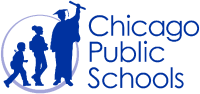# CPS Volunteer Requirements

According to CPS policy, anybody who is going to be in contact with students must be approved as a CPS volunteer. There are two levels of volunteers, Level 1 and Level 2.

## Level I Volunteers

(Please allow 8-12 weeks for the entire process)
A fingerprint criminal background check is required for all Level I Volunteers.

A Level I volunteer is:

1. A parent providing volunteer service in their child’s school or classroom for 10 or more hours/week;
2. An individual providing volunteer service for 5 or more hours/week at a school where s/he does not have a child enrolled;
3. Any individual chaperoning an overnight school-sponsored trip, regardless of the hours/week that the volunteer serves (See also the Student Travel Policy).
 1 A parent providing volunteer service in their child’s school or classroom for 10 or more hours/week;
 2 An individual providing volunteer service for 5 or more hours/week at a school where s/he does not have a child enrolled;
 3 Any individual chaperoning an overnight school-sponsored trip, regardless of the hours/week that the volunteer serves (See also the Student Travel Policy).

## Level II Volunteers

(This process can be completed in a day & is required even of volunteers for Friends of Northside events)
A fingerprint criminal background check is not required for Level II Volunteers.

A Level II Volunteer is:

1. A parent providing supervised volunteer service in their child’s school or classroom for less than 10 hours/week;
2. Any individual providing volunteer service for less than 5 hours/week at a school where s/he does not have a child enrolled;
3. An individual providing incidental volunteer service with no ongoing individualized interaction with a student(s) including those who speak at a class/assembly, judge academic competitions, give musical performance, participate in the “Principal for a Day” program, job shadowing event or other one-time event provided where there is direct supervision of the activity/event by regular school employees;
4. A parent accompanying his/her child’s class on a one-day field trip or another type of extracurricular activity that does not involve an overnight stay;
5. An individual providing volunteer service on projects/activities involving no or nominal contact with children (home-based volunteer activities, volunteers serving at Central or Network office).
 1 A parent providing supervised volunteer service in their child’s school or classroom for less than 10 hours/week;
 2 Any individual providing volunteer service for less than 5 hours/week at a school where s/he does not have a child enrolled;
 3 An individual providing incidental volunteer service with no ongoing individualized interaction with a student(s) including those who speak at a class/assembly, judge academic competitions, give musical performance, participate in the “Principal for a Day” program, job shadowing event or other one-time event provided where there is direct supervision of the activity/event by regular school employees;
 4 A parent accompanying his/her child’s class on a one-day field trip or another type of extracurricular activity that does not involve an overnight stay;
 5 An individual providing volunteer service on projects/activities involving no or nominal contact with children (home-based volunteer activities, volunteers serving at Central or Network office).

To become a volunteer, please visit CPS' Volunteer website and follow the instructions. There is also a link on Northside's website on the Parents page Northside Volunteers.

Assistant Principal, Kaitlin O'Hare is Northside's Volunteer Coordinator. After applying to become a volunteer, you must visit the school and show Ms. O'Hare your ID, so she can approve you to move forward in the volunteer process. For Level II volunteers, that is the only step you must take. For Level 1, you will receive further instructions from CPS.

 table div table+table+table+table+table+table+table+table+table+table+table+table+table+table+table div table{width:100%;padding:0}table div table+table+table+table+table+table+table+table+table+table+table+table+table+table+table div table img{width:96.23%;padding:0;float:none}table div table+table+table+table+table+table+table+table+table+table+table+table+table+table+table div table td{width:100%;padding:0 1.88% 18px}/* styles */# This Week in Sports

Come out and support our student athletes!

## Women's Tennis

▪ Monday, September 16, 5:00 pm (V) vs. Lane Tech at McFetridge Park
▪ Tuesday, September 17, 5:00 pm (V) vs. Lake View at NCP
▪ Thursday, September 19, 5:00 pm (V) vs. Jones College Prep at Roosevelt Park
 ▪ Monday, September 16, 5:00 pm (V) vs. Lane Tech at McFetridge Park
 ▪ Tuesday, September 17, 5:00 pm (V) vs. Lake View at NCP
 ▪ Thursday, September 19, 5:00 pm (V) vs. Jones College Prep at Roosevelt Park

## Men's Soccer

▪ Monday, September 16, 4:30 pm (V) and 6:00 pm (JV) vs. Jones College Prep at Jones College Prep
▪ Wednesday, September 18, 4:30 pm (V) and 6:00 pm (JV) vs. Taft at NCP
▪ Thursday, September 19, 5:00 pm (S) vs. Lincoln Park at NCP
 ▪ Monday, September 16, 4:30 pm (V) and 6:00 pm (JV) vs. Jones College Prep at Jones College Prep
 ▪ Wednesday, September 18, 4:30 pm (V) and 6:00 pm (JV) vs. Taft at NCP
 ▪ Thursday, September 19, 5:00 pm (S) vs. Lincoln Park at NCP

## Women's Volleyball

▪ Tuesday, September 17, 5:00 pm (V) and 6:00 pm (JV) vs. Von Steuben at NCP
▪ Thursday, September 19, 5:00 pm (V) and 6:00 pm (JV) vs. Jones College Prep at Jones College Prep
▪ Friday, September 20, 4:30 pm (F), 5:30 pm (JV), and 6:30 pm (V) vs. University at University High School
 ▪ Tuesday, September 17, 5:00 pm (V) and 6:00 pm (JV) vs. Von Steuben at NCP
 ▪ Thursday, September 19, 5:00 pm (V) and 6:00 pm (JV) vs. Jones College Prep at Jones College Prep
 ▪ Friday, September 20, 4:30 pm (F), 5:30 pm (JV), and 6:30 pm (V) vs. University at University High School

## Women's Softball

▪ Monday, September 16, 4:00 pm (V) vs. Lane Tech at Horner Park
▪ Thursday, September 19, 4:30 pm (V) vs. Lincoln Park at NCP
 ▪ Monday, September 16, 4:00 pm (V) vs. Lane Tech at Horner Park
 ▪ Thursday, September 19, 4:30 pm (V) vs. Lincoln Park at NCP

## Women's Swimming

▪ Thursday, September 19, 5:00 pm (V) vs. Lane Tech at Lane Tech High School
 ▪ Thursday, September 19, 5:00 pm (V) vs. Lane Tech at Lane Tech High School

## Women's Golf

▪ Wednesday, September 18, 3:00 pm (V) vs. Taft, Lane Tech at Billy Caldwell Golf Course
▪ Saturday, September 21, 11:00 am (V) vs. TBA at Jackson Park Golf Course
 ▪ Wednesday, September 18, 3:00 pm (V) vs. Taft, Lane Tech at Billy Caldwell Golf Course
 ▪ Saturday, September 21, 11:00 am (V) vs. TBA at Jackson Park Golf Course

## Men's Golf

▪ Tuesday, September 17, 3:30 pm (V) vs. Disney II Magnet, Whitney Young at Marovitz Golf Course
▪ Thursday, September 19, 3:30 pm (V) vs. Jones College Prep, Lincoln Park, Walter Payton College Prep at Billy Caldwell Golf Course
▪ Saturday, September 21, 11:00 am (V) vs. TBA at Jackson Park Golf Course
 ▪ Tuesday, September 17, 3:30 pm (V) vs. Disney II Magnet, Whitney Young at Marovitz Golf Course
 ▪ Thursday, September 19, 3:30 pm (V) vs. Jones College Prep, Lincoln Park, Walter Payton College Prep at Billy Caldwell Golf Course
 ▪ Saturday, September 21, 11:00 am (V) vs. TBA at Jackson Park Golf Course

## Women's Cross Country

▪ Tuesday, September 17, 4:30 pm (V) vs. Mather at NCP
 ▪ Tuesday, September 17, 4:30 pm (V) vs. Mather at NCP

## Men's Cross Country

▪ Tuesday, September 17, 4:30 pm (V) vs. Mather at NCP
 ▪ Tuesday, September 17, 4:30 pm (V) vs. Mather at NCP
 /* styles */ Visit the NCP Athletics website for additional information about Northside teams.
 table div table+table+table+table+table+table+table+table+table+table+table+table+table+table+table+table+table+table div table{width:100%;padding:0}table div table+table+table+table+table+table+table+table+table+table+table+table+table+table+table+table+table+table div table img{width:96.23%;padding:0;float:none}table div table+table+table+table+table+table+table+table+table+table+table+table+table+table+table+table+table+table div table td{width:100%;padding:0 1.88% 18px}/* styles */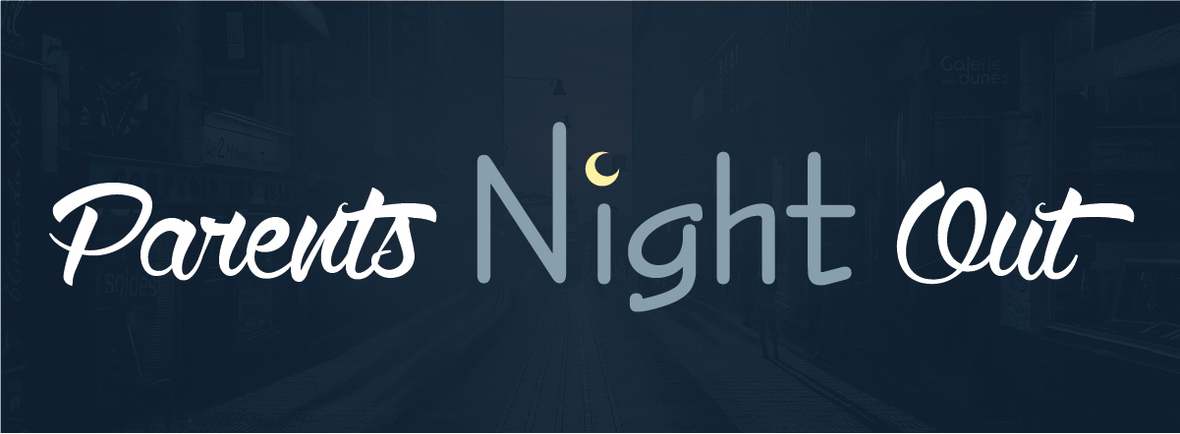# Fall Parents Night Out

Thursday, September 26
6:00 pm
Via Veneto
6340 N. Lincoln Ave

Join us for a Parents' Night Out at Via Veneto. There will be complimentary appetizers and a cash bar. Catch up with old friends and make new ones! Please sign up HERE so we can let the restaurant know how many people to expect.

 table div table+table+table+table+table+table+table+table+table+table+table+table+table+table+table+table+table+table+table+table+table div table{width:100%;padding:0}table div table+table+table+table+table+table+table+table+table+table+table+table+table+table+table+table+table+table+table+table+table div table img{width:96.23%;padding:0;float:none}table div table+table+table+table+table+table+table+table+table+table+table+table+table+table+table+table+table+table+table+table+table div table td{width:100%;padding:0 1.88% 18px}/* styles */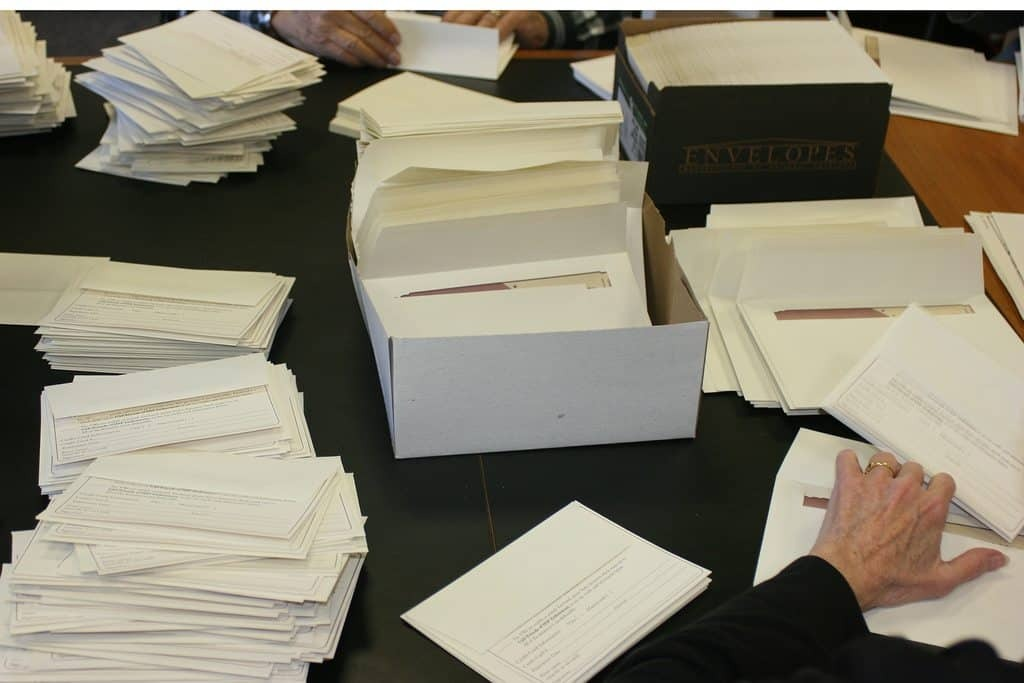# Annual Appeal Mailing

Wednesday, October 2
4:15 - 5:30 pm

We're kicking off our Annual Appeal and need 10 volunteers to label and stuff envelopes for the mailing that goes to all NCP families and friends. No skills are required and it's guaranteed to be a good time! To sign up, click HERE.

 table div table+table+table+table+table+table+table+table+table+table+table+table+table+table+table+table+table+table+table+table+table+table+table div table{width:100%;padding:0}table div table+table+table+table+table+table+table+table+table+table+table+table+table+table+table+table+table+table+table+table+table+table+table div table img{width:96.23%;padding:0;float:none}table div table+table+table+table+table+table+table+table+table+table+table+table+table+table+table+table+table+table+table+table+table+table+table div table td{width:100%;padding:0 1.88% 18px}/* styles */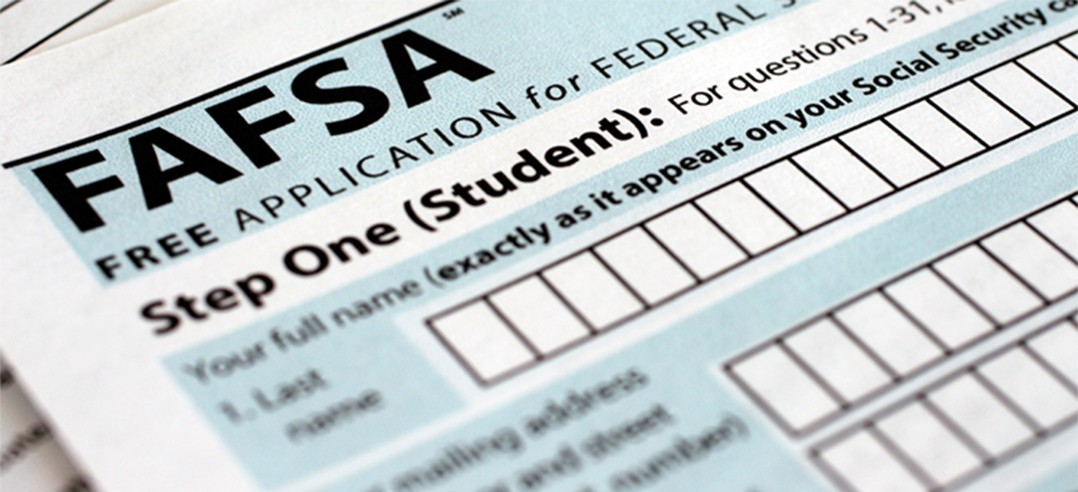# Financial Aid Completion Workshops

Tuesday, October 15
6:00 - 8:00 pm

The counseling department will be offering FAFSA Completion Workshops for English and Spanish speaking parents and students on Tuesday, October 15 from 6:00 - 8:00 pm. Additional student workshops will be held during the day for students who would like to start their FAFSA as soon as the application becomes available or if they would like one on one support.

Students can register for the sessions offered during the school day in their Enriching Student Portal.

## Presentations

ISAC Student FAFSA Workshop
Wednesday, October 2
Room 224
Two sessions: 1:25 - 2:10 pm and 2:15 - 3:00 pm

ISAC Parent and Student FAFSA Workshop (English & Spanish)
Tuesday, October 16
6:00 - 8:00 pm
Room 219

ISAC Student Financial Aid Follow-up
Wednesday, November 20
Room 224
Two sessions: 1:25 - 2:10 pm and 2:15 - 3:00 pm

 table div table+table+table+table+table+table+table+table+table+table+table+table+table+table+table+table+table+table+table+table+table+table+table+table+table div table{width:100%;padding:0}table div table+table+table+table+table+table+table+table+table+table+table+table+table+table+table+table+table+table+table+table+table+table+table+table+table div table img{width:96.23%;padding:0;float:none}table div table+table+table+table+table+table+table+table+table+table+table+table+table+table+table+table+table+table+table+table+table+table+table+table+table div table td{width:100%;padding:0 1.88% 18px}/* styles */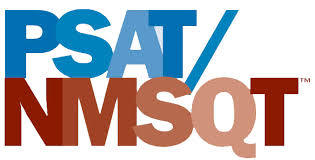# PSAT/NMST Exam

Wednesday, October 16
8:00 am

Northside College Prep sophomores and juniors will participate in the PSAT/NMSQT exam. On the day of the exam, students are expected to report to school no later than 8:00 am for a full day of school. More details about the exam will be shared with students and parents in the near future.

 table div table+table+table+table+table+table+table+table+table+table+table+table+table+table+table+table+table+table+table+table+table+table+table+table+table+table+table div table{width:100%;padding:0}table div table+table+table+table+table+table+table+table+table+table+table+table+table+table+table+table+table+table+table+table+table+table+table+table+table+table+table div table img{width:96.23%;padding:0;float:none}table div table+table+table+table+table+table+table+table+table+table+table+table+table+table+table+table+table+table+table+table+table+table+table+table+table+table+table div table td{width:100%;padding:0 1.88% 18px}/* styles */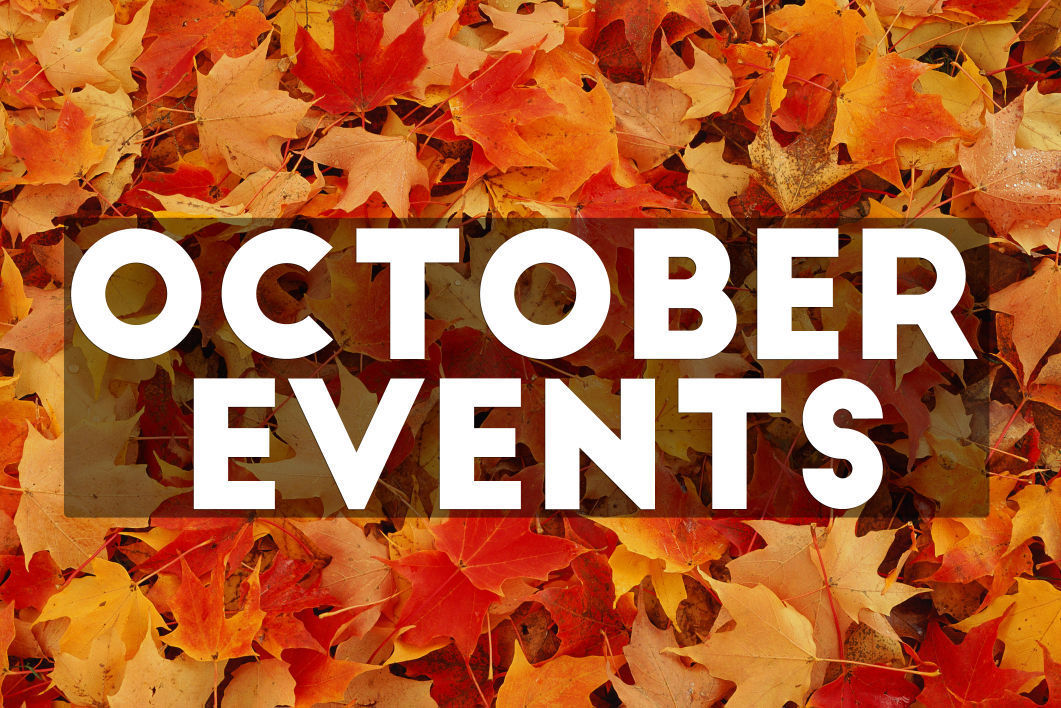# Important October Dates

▪ Wednesday, October 2 - Student FAFSA Workshop
▪ Friday, October 4 - First Friday
▪ Monday, October 14 - Columbus Day (no school)
▪ Tuesday, October 15 - LSC Meeting & FAFSA Completion Workshop
▪ Wednesday, October 16 - PSAT/NMST Exam
▪ Friday, October 18 - Latino Fest
▪ Tuesday, October 22 - FON Meeting
▪ Friday, October 25 - Halloween Dance (sponsored by the Latin Club)
▪ Sunday, October 27 - NCP Open House
▪ Tuesday, October 29 - Halloween Concert
 ▪ Wednesday, October 2 - Student FAFSA Workshop
 ▪ Friday, October 4 - First Friday
 ▪ Monday, October 14 - Columbus Day (no school)
 ▪ Tuesday, October 15 - LSC Meeting & FAFSA Completion Workshop
 ▪ Wednesday, October 16 - PSAT/NMST Exam
 ▪ Friday, October 18 - Latino Fest
 ▪ Tuesday, October 22 - FON Meeting
 ▪ Friday, October 25 - Halloween Dance (sponsored by the Latin Club)
 ▪ Sunday, October 27 - NCP Open House
 ▪ Tuesday, October 29 - Halloween Concert
 table div table+table+table+table+table+table+table+table+table+table+table+table+table+table+table+table+table+table+table+table+table+table+table+table+table+table+table+table+table+table div table{width:100%;padding:0}table div table+table+table+table+table+table+table+table+table+table+table+table+table+table+table+table+table+table+table+table+table+table+table+table+table+table+table+table+table+table div table img{width:96.23%;padding:0;float:none}table div table+table+table+table+table+table+table+table+table+table+table+table+table+table+table+table+table+table+table+table+table+table+table+table+table+table+table+table+table+table div table td{width:100%;padding:0 1.88% 18px}/* styles */# No Experience Necessary

Make a commitment to Northside by offering your time for any of these rewarding on-going volunteer positions. Email Friends of Northside to indicate your interest or ask questions.

## Graphic Designer

Provide professional guidance and materials for various design needs throughout the year.

## Volunteer Coordinator

Manage and update our online volunteer system as needed throughout the year.

## Help Needed with the Teacher's Lounge

We are looking for one additional helper to keep the Teachers' Lounge stocked with coffee, tea, etc. We're looking for someone who can come on Wednesdays. It takes about 30 minutes and the time is flexible. It's a great way to get involved in your child's school! Contact Eileen at 617.642.6267 for details.

## Spring Benefit Committee

The Spring Benefit is NCP’s major spring fundraiser. Get involved, get to know NCP staff and parents, and make a profound impact on Northside College Prep.

When is the Spring Benefit?
Friday, March 13, 2020

What’s the time commitment for the team?

What roles are available?
* Entertainment Chair--one or two people to determine and arrange the entertainment for the Spring Benefit.
* Auction Item Solicitors--at least five people to solicit auction items.
* Printed Materials Chair--one or two people to design and produce all the printed materials.

 table div table+table+table+table+table+table+table+table+table+table+table+table+table+table+table+table+table+table+table+table+table+table+table+table+table+table+table+table+table+table+table+table+table+table div table{width:100%;padding:0}table div table+table+table+table+table+table+table+table+table+table+table+table+table+table+table+table+table+table+table+table+table+table+table+table+table+table+table+table+table+table+table+table+table+table div table img{width:96.23%;padding:0;float:none}table div table+table+table+table+table+table+table+table+table+table+table+table+table+table+table+table+table+table+table+table+table+table+table+table+table+table+table+table+table+table+table+table+table+table div table td{width:100%;padding:0 1.88% 18px}/* styles */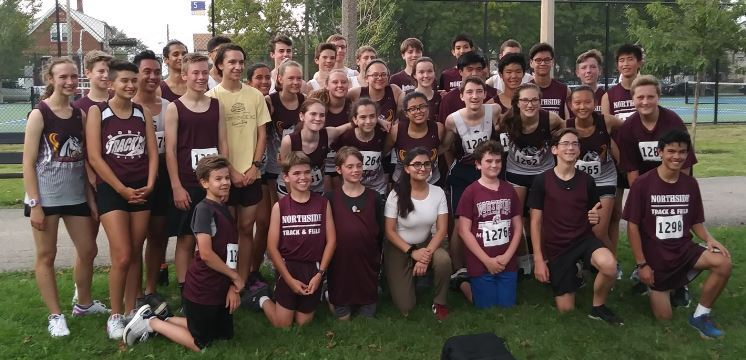# Cross Country Teams Takes 1st in Fall Frolic

The Northside cross country teams competed in the Steinmetz Fall Frolic meet at Riis Park on Wednesday, placing first out of nine teams in the women’s race, with 27 points, and first out of 15 in the men’s race, with 57 points. A total of 47 Mustangs competed in the two-mile race.

Elia Ton-That (So.) and Gwen Casey (Sr.) went one and two in the women’s race after leading from start to finish. Maria Avina (Jr.), Nora Hill (So.), and Allison Feeney (So.) rounded out the Mustang’s top-five, placing 10th, 11th, and 12th in the race for a total of 27 points. In addition to the five scoring runners, three more Northsiders finished among the top-25, Medina Karic (Jr.), Kate Clemenz (Sr.), and Joselyn Santos (Sr.).

Ezra Hill (Jr.) came from behind in the men’s race to capture first place in a close finish. Seth Karall (Sr.), Maciej Zychowski (Jr.), Liam Pentangelo (Fr.), and Arlo Johnston (Fr.) placed 6th, 14th, 15th, and 21st to complete the team’s top-five. Addison Morley (Fr.) also finished in the top-25.

The teams have a series of Tuesday meets, September 17, 24, and October 1, hosted by Mather at Legion Park.

# Do you have news or team results to share with the Northside parent community?

 table div table+table+table+table+table+table+table+table+table+table+table+table+table+table+table+table+table+table+table+table+table+table+table+table+table+table+table+table+table+table+table+table+table+table+table+table+table+table+table div table{width:100%;padding:0}table div table+table+table+table+table+table+table+table+table+table+table+table+table+table+table+table+table+table+table+table+table+table+table+table+table+table+table+table+table+table+table+table+table+table+table+table+table+table+table div table img{width:96.23%;padding:0;float:none}table div table+table+table+table+table+table+table+table+table+table+table+table+table+table+table+table+table+table+table+table+table+table+table+table+table+table+table+table+table+table+table+table+table+table+table+table+table+table+table div table td{width:100%;padding:0 1.88% 18px}/* styles */# September College Visits at Northside

Monday, September 16
University of California, Santa Cruz, 9:48 am
University of St. Francis, 9:48 am
Syracuse University, 9:48 am
Sarah Lawrence College, 11:36 am
Stetson University, 11:36 am
Case Western Reserve University, 12:26 pm
Emory University, 12:26 pm
University of Michigan, 3:15 pm

Tuesday, September 17
Columbia College Chicago, 9:48 am
Hillsdale College, 9:48 am
College of the Holy Cross, 9:48 am
Saint Mary's College, 9:48 am
Columbia University International Dual BA Programs, 11:36 am
Tufts University, 11:36 am
University of Aberdeen, 12:26 pm
University of Southern California, 3:15 pm

Wednesday, September 18
University of Illinois at Urbana-Champaign, 10:40 am
Chicago State University, 11:20 pm
IE University - Segovia, 11:20 am
Chicago State University, 12:40 pm
Louisiana State University, 12:40 pm
The College of Wooster, 12:40 pm
John Carroll University, 3:15 pm

Thursday, September 19
Southern Illinois University, Carbondale, 9:48 am
Washington University in St. Louis, 9:48 am
Knox College, 10:38 am
University of Notre Dame, 10:38 am
Temple University Japan, 12:26 pm
Michigan State University, 3:15 pm

Friday, September 20
Reed College, 9:48 am
Rollins College, 9:48 am
Boston University, 11:36 am
Southern Illinois University Edwardsville, 12:26 pm

Monday, September 23
Massachusetts Institute of Technology, 3:15 pm

Tuesday, September 24
St. John's University, 10:38 am
Saint Louis University, 12:26 pm

Wednesday, September 25
Suffolk University, 11:20 am
Kalamazoo College, 1:25 pm
Indiana University at Bloomington, 3:15 pm

Thursday, September 26
DePauw University, 9:48 am
Missouri State University, 12:26 pm
American University, 3:15 pm

Monday, September 30
Barnard College, 9:48 am
University of California, Berkeley, 9:48 am
Middlebury College, 9:48 am
Wesleyan University, 10:38 am
Lehigh University, 11:36 am
Temple University, 3:15 pm

The list of college visits is updated frequently. For the most current information, parents of juniors and seniors should log into their parent account for Naviance.

Students are highly encouraged to meet with the reps who take the time to visit Northside. Oftentimes these are the same regional reps who will be reading your child's application! Students may ask a teacher to be briefly excused from class to meet a college representative.

 table div table+table+table+table+table+table+table+table+table+table+table+table+table+table+table+table+table+table+table+table+table+table+table+table+table+table+table+table+table+table+table+table+table+table+table+table+table+table+table+table+table+table div table{width:100%;padding:0}table div table+table+table+table+table+table+table+table+table+table+table+table+table+table+table+table+table+table+table+table+table+table+table+table+table+table+table+table+table+table+table+table+table+table+table+table+table+table+table+table+table+table div table img{width:96.23%;padding:0;float:none}table div table+table+table+table+table+table+table+table+table+table+table+table+table+table+table+table+table+table+table+table+table+table+table+table+table+table+table+table+table+table+table+table+table+table+table+table+table+table+table+table+table+table div table td{width:100%;padding:0 1.88% 18px}/* styles */# CPS Scholarship Notifications and College Events Around the City

For more details about the upcoming events and to access the most recent updates, view the College Calendar on the Northside Prep Counseling Website HERE.

 table div table+table+table+table+table+table+table+table+table+table+table+table+table+table+table+table+table+table+table+table+table+table+table+table+table+table+table+table+table+table+table+table+table+table+table+table+table+table+table+table+table+table+table+table div table{width:100%;padding:0}table div table+table+table+table+table+table+table+table+table+table+table+table+table+table+table+table+table+table+table+table+table+table+table+table+table+table+table+table+table+table+table+table+table+table+table+table+table+table+table+table+table+table+table+table div table img{width:96.23%;padding:0;float:none}table div table+table+table+table+table+table+table+table+table+table+table+table+table+table+table+table+table+table+table+table+table+table+table+table+table+table+table+table+table+table+table+table+table+table+table+table+table+table+table+table+table+table+table+table div table td{width:100%;padding:0 1.88% 18px}/* styles */# New to Parent Portal?

1. Obtain your student's PIN, which should be on your acceptance letter or school welcome letter.
2. Create an account HERE.
 1 Obtain your student's PIN, which should be on your acceptance letter or school welcome letter.
 2 Create an account HERE.

PINs can only be provided to the parent/guardian in person or by mail to the address on file. They cannot be provided by phone or email, or to a student. To request your PIN, please contact Sonia Fernandez in the Counseling Office.

 table div table+table+table+table+table+table+table+table+table+table+table+table+table+table+table+table+table+table+table+table+table+table+table+table+table+table+table+table+table+table+table+table+table+table+table+table+table+table+table+table+table+table+table+table+table+table div table{width:100%;padding:0}table div table+table+table+table+table+table+table+table+table+table+table+table+table+table+table+table+table+table+table+table+table+table+table+table+table+table+table+table+table+table+table+table+table+table+table+table+table+table+table+table+table+table+table+table+table+table div table img{width:96.23%;padding:0;float:none}table div table+table+table+table+table+table+table+table+table+table+table+table+table+table+table+table+table+table+table+table+table+table+table+table+table+table+table+table+table+table+table+table+table+table+table+table+table+table+table+table+table+table+table+table+table+table div table td{width:100%;padding:0 1.88% 18px}/* styles */# FON Connect

Friends of Northside uses a customer/constituent relationship management (CRM) system known as FON Connect that allows our parent volunteer organization to work from one database for events. It is not a CPS database and is completely different and separate from the CPS Parent Portal and Northside's EPay website.

FON Connect also serves as the NCP Family Directory and allows users to review their donation history, update their contact information, register for upcoming events, and more. Each parent has a separate account. Transactions, including donations, are recorded in the account of the parent who initiated the transaction or donation.

If you’re new to FON Connect, you'll need to set up a login and password. To do so:

 table div table+table+table+table+table+table+table+table+table+table+table+table+table+table+table+table+table+table+table+table+table+table+table+table+table+table+table+table+table+table+table+table+table+table+table+table+table+table+table+table+table+table+table+table+table+table+table+table div table{width:100%;padding:0}table div table+table+table+table+table+table+table+table+table+table+table+table+table+table+table+table+table+table+table+table+table+table+table+table+table+table+table+table+table+table+table+table+table+table+table+table+table+table+table+table+table+table+table+table+table+table+table+table div table img{width:96.23%;padding:0;float:none}table div table+table+table+table+table+table+table+table+table+table+table+table+table+table+table+table+table+table+table+table+table+table+table+table+table+table+table+table+table+table+table+table+table+table+table+table+table+table+table+table+table+table+table+table+table+table+table+table div table td{width:100%;padding:0 1.88% 18px}/* styles */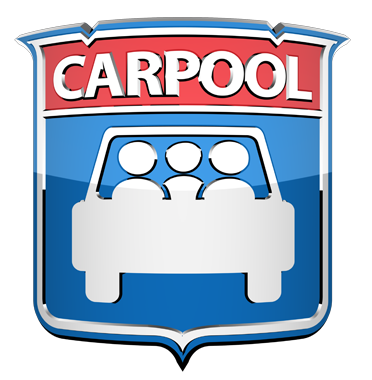# NCP Carpool Connection!

If you're interested in carpooling to school, add your address to the 2019-2020 NCP Carpool map and reach out to contact a potential carpool family on the map. Find the map HERE or on the Welcome page of FON Connect.

# Attention, Parents of CTA Commuters

Did you know that the #82 and #92 buses pick up students in front of Northside at the end of the school day? The Kimball Brown line station is also close to school at Kimball and Lawrence.

These are just a few of the ways students can use public transportation to commute to school. More information on routes for your student can be found at the CTA website HERE.

 table div table+table+table+table+table+table+table+table+table+table+table+table+table+table+table+table+table+table+table+table+table+table+table+table+table+table+table+table+table+table+table+table+table+table+table+table+table+table+table+table+table+table+table+table+table+table+table+table+table+table+table div table{width:100%;padding:0}table div table+table+table+table+table+table+table+table+table+table+table+table+table+table+table+table+table+table+table+table+table+table+table+table+table+table+table+table+table+table+table+table+table+table+table+table+table+table+table+table+table+table+table+table+table+table+table+table+table+table+table div table img{width:96.23%;padding:0;float:none}table div table+table+table+table+table+table+table+table+table+table+table+table+table+table+table+table+table+table+table+table+table+table+table+table+table+table+table+table+table+table+table+table+table+table+table+table+table+table+table+table+table+table+table+table+table+table+table+table+table+table+table div table td{width:100%;padding:0 1.88% 18px}/* styles */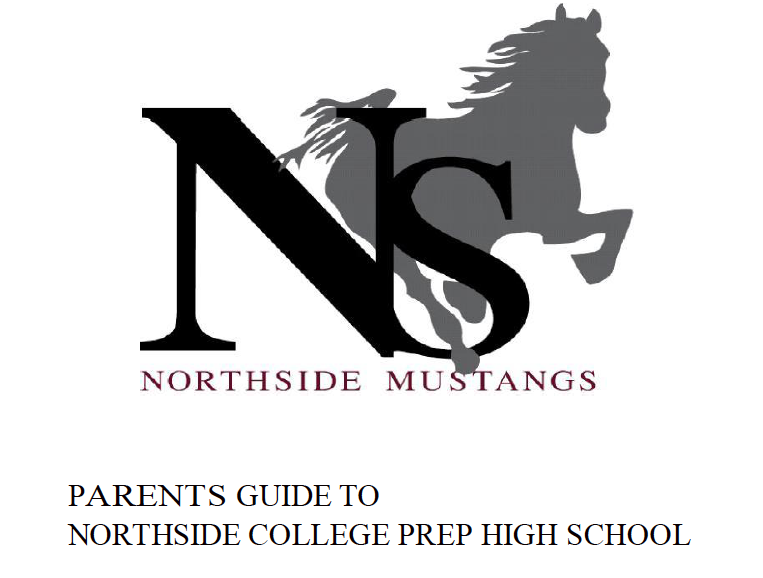# Navigating Northside

Navigating Northside, our parent handbook, is available on the school website under the "Parents" tab. Click HERE to access it directly.

 table div table+table+table+table+table+table+table+table+table+table+table+table+table+table+table+table+table+table+table+table+table+table+table+table+table+table+table+table+table+table+table+table+table+table+table+table+table+table+table+table+table+table+table+table+table+table+table+table+table+table+table+table+table div table{width:100%;padding:0}table div table+table+table+table+table+table+table+table+table+table+table+table+table+table+table+table+table+table+table+table+table+table+table+table+table+table+table+table+table+table+table+table+table+table+table+table+table+table+table+table+table+table+table+table+table+table+table+table+table+table+table+table+table div table img{width:96.23%;padding:0;float:none}table div table+table+table+table+table+table+table+table+table+table+table+table+table+table+table+table+table+table+table+table+table+table+table+table+table+table+table+table+table+table+table+table+table+table+table+table+table+table+table+table+table+table+table+table+table+table+table+table+table+table+table+table+table div table td{width:100%;padding:0 1.88% 18px}/* styles */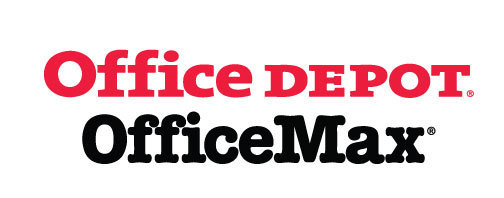# Give Back to Northside

Purchase school and office supplies at Office Depot and OfficeMax and Northside can receive 5% back in credits or FREE supplies. Simply provide our school ID 70031171 at checkout, online, or in stores. There’s no cost to you!

 table div table+table+table+table+table+table+table+table+table+table+table+table+table+table+table+table+table+table+table+table+table+table+table+table+table+table+table+table+table+table+table+table+table+table+table+table+table+table+table+table+table+table+table+table+table+table+table+table+table+table+table+table+table+table+table+table div table{width:100%;padding:0}table div table+table+table+table+table+table+table+table+table+table+table+table+table+table+table+table+table+table+table+table+table+table+table+table+table+table+table+table+table+table+table+table+table+table+table+table+table+table+table+table+table+table+table+table+table+table+table+table+table+table+table+table+table+table+table+table div table img{width:96.23%;padding:0;float:none}table div table+table+table+table+table+table+table+table+table+table+table+table+table+table+table+table+table+table+table+table+table+table+table+table+table+table+table+table+table+table+table+table+table+table+table+table+table+table+table+table+table+table+table+table+table+table+table+table+table+table+table+table+table+table+table+table div table td{width:100%;padding:0 1.88% 18px}/* styles */table.module-56{width:66.42%;padding:0}table div table+table+table+table+table+table+table+table+table+table+table+table+table+table+table+table+table+table+table+table+table+table+table+table+table+table+table+table+table+table+table+table+table+table+table+table+table+table+table+table+table+table+table+table+table+table+table+table+table+table+table+table+table+table+table+table+table div table{width:66.42%;float:none;margin-left:auto;margin-right:auto;padding:0}table div table+table+table+table+table+table+table+table+table+table+table+table+table+table+table+table+table+table+table+table+table+table+table+table+table+table+table+table+table+table+table+table+table+table+table+table+table+table+table+table+table+table+table+table+table+table+table+table+table+table+table+table+table+table+table+table+table div table a{border:0 none;text-decoration:none}table div table+table+table+table+table+table+table+table+table+table+table+table+table+table+table+table+table+table+table+table+table+table+table+table+table+table+table+table+table+table+table+table+table+table+table+table+table+table+table+table+table+table+table+table+table+table+table+table+table+table+table+table+table+table+table+table+table div table img{width:100%!important;border:0 none;text-decoration:none}table div table+table+table+table+table+table+table+table+table+table+table+table+table+table+table+table+table+table+table+table+table+table+table+table+table+table+table+table+table+table+table+table+table+table+table+table+table+table+table+table+table+table+table+table+table+table+table+table+table+table+table+table+table+table+table+table+table div table td{width:100%;padding:0}/* styles */

# Support Northside - Shop AmazonSmile

AmazonSmile is a simple and automatic way to support Northside every time you shop.

Please note that our AmazonSmile name is Friends of "North" "Side" (North Side - two words at AmazonSmile).

 table div table+table+table+table+table+table+table+table+table+table+table+table+table+table+table+table+table+table+table+table+table+table+table+table+table+table+table+table+table+table+table+table+table+table+table+table+table+table+table+table+table+table+table+table+table+table+table+table+table+table+table+table+table+table+table+table+table+table+table div table{width:100%;padding:0}table div table+table+table+table+table+table+table+table+table+table+table+table+table+table+table+table+table+table+table+table+table+table+table+table+table+table+table+table+table+table+table+table+table+table+table+table+table+table+table+table+table+table+table+table+table+table+table+table+table+table+table+table+table+table+table+table+table+table+table div table img{width:96.23%;padding:0;float:none}table div table+table+table+table+table+table+table+table+table+table+table+table+table+table+table+table+table+table+table+table+table+table+table+table+table+table+table+table+table+table+table+table+table+table+table+table+table+table+table+table+table+table+table+table+table+table+table+table+table+table+table+table+table+table+table+table+table+table+table div table td{width:100%;padding:0 1.88% 18px}/* styles */# Follow Us on Social Media

▪ Northside Prep Parents Facebook Page, a closed group for parents, by parents, to share their knowledge of Northside
▪ Friends of Northside Facebook Page, for news everyone can view
 ▪ Northside Prep Parents Facebook Page, a closed group for parents, by parents, to share their knowledge of Northside
 ▪ Friends of Northside Facebook Page, for news everyone can view
 table div table+table+table+table+table+table+table+table+table+table+table+table+table+table+table+table+table+table+table+table+table+table+table+table+table+table+table+table+table+table+table+table+table+table+table+table+table+table+table+table+table+table+table+table+table+table+table+table+table+table+table+table+table+table+table+table+table+table+table+table+table div table{width:100%;padding:0}table div table+table+table+table+table+table+table+table+table+table+table+table+table+table+table+table+table+table+table+table+table+table+table+table+table+table+table+table+table+table+table+table+table+table+table+table+table+table+table+table+table+table+table+table+table+table+table+table+table+table+table+table+table+table+table+table+table+table+table+table+table div table img{width:96.23%;padding:0;float:none}table div table+table+table+table+table+table+table+table+table+table+table+table+table+table+table+table+table+table+table+table+table+table+table+table+table+table+table+table+table+table+table+table+table+table+table+table+table+table+table+table+table+table+table+table+table+table+table+table+table+table+table+table+table+table+table+table+table+table+table+table+table div table td{width:100%;padding:0 1.88% 18px}/* styles */Northside’s website can be found HERE.

Friends of Northside's website can be found HERE.

 table div table+table+table+table+table+table+table+table+table+table+table+table+table+table+table+table+table+table+table+table+table+table+table+table+table+table+table+table+table+table+table+table+table+table+table+table+table+table+table+table+table+table+table+table+table+table+table+table+table+table+table+table+table+table+table+table+table+table+table+table+table+table+table div table{width:100%;padding:0}table div table+table+table+table+table+table+table+table+table+table+table+table+table+table+table+table+table+table+table+table+table+table+table+table+table+table+table+table+table+table+table+table+table+table+table+table+table+table+table+table+table+table+table+table+table+table+table+table+table+table+table+table+table+table+table+table+table+table+table+table+table+table+table div table img{width:96.23%;padding:0;float:none}table div table+table+table+table+table+table+table+table+table+table+table+table+table+table+table+table+table+table+table+table+table+table+table+table+table+table+table+table+table+table+table+table+table+table+table+table+table+table+table+table+table+table+table+table+table+table+table+table+table+table+table+table+table+table+table+table+table+table+table+table+table+table+table div table td{width:100%;padding:0 1.88% 18px}/* styles */